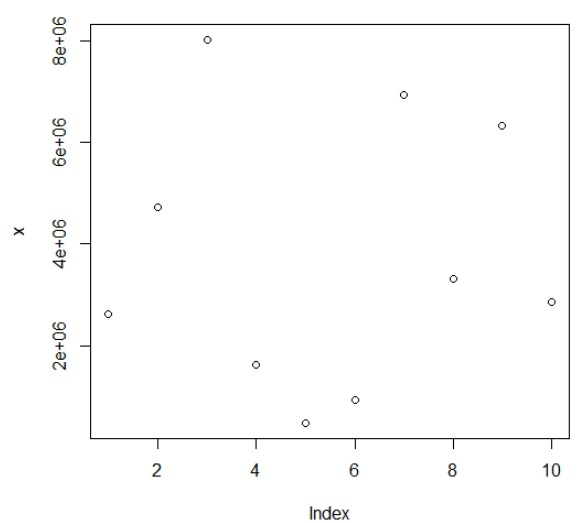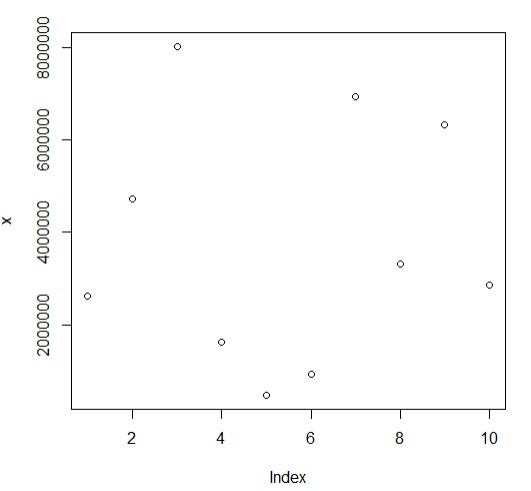# How to remove scientific notation form base R plot?

R ProgrammingServer Side ProgrammingProgramming

To remove scientific notation form base R plot, we can follow the below steps −

• First of all, create a vector and its plot using plot function.
• Then, use options(scipen=999) to remove scientific notation from the plot.

## Create the vector and plot

Using sample function to create a vector and plot the vector with plot function −

Live Demo

> x<-sample(1:10000000,10)
> plot(x)

## Output## Remove the scientific notation from the plot

Use options(scipen=999) function and again create the same plot −

Live Demo

> x<-sample(1:10000000,10)
> options(scipen=999)
> plot(x)

## Output